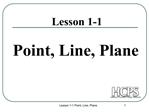DownloadDownload PresentationLesson 1-1 Point, Line, Plane

# Lesson 1-1 Point, Line, Plane

Télécharger la présentation## Lesson 1-1 Point, Line, Plane

- - - - - - - - - - - - - - - - - - - - - - - - - - - E N D - - - - - - - - - - - - - - - - - - - - - - - - - - -
##### Presentation Transcript

1. Lesson 1-1 Point, Line, Plane 1 Lesson 1-1 Point, Line, Plane

2. Lesson 1-1 Point, Line, Plane 2

3. Lesson 1-1 Point, Line, Plane 3 Lines Lines extend indefinitely and have no thickness or width. How to sketch : using arrows at both ends. How to name: 2 ways (1) small script letter � line n (2) any two points on the line - Never name a line using three points -

4. Lesson 1-1 Point, Line, Plane 4 Collinear Points Collinear points are points that lie on the same line. (The line does not have to be visible.) A point lies on the line if the coordinates of the point satisfy the equation of the line. Ex: To find if A (1, 0) is collinear with the points on the line y = -3x + 3. Substitute x = 1 and y = 0 in the equation. 0 = -3 (1) + 3 0 = -3 + 3 0 = 0 The point A satisfies the equation, therefore the point is collinear with the points on the line.

5. Lesson 1-1 Point, Line, Plane 5 Planes A plane is a flat surface that extends indefinitely in all directions. How to sketch: Use a parallelogram (four sided figure) How to name: 2 ways (1) Capital script letter � Plane M (2) Any 3 non collinear points in the plane - Plane: ABC/ ACB / BAC / BCA / CAB / CBA

6. Lesson 1-1 Point, Line, Plane 6 Different planes in a figure:

7. Lesson 1-1 Point, Line, Plane 7 Other planes in the same figure: Any three non collinear points determine a plane!

8. Lesson 1-1 Point, Line, Plane 8 Coplanar Objects Coplanar objects (points, lines, etc.) are objects that lie on the same plane. The plane does not have to be visible.

9. Lesson 1-1 Point, Line, Plane 9 Intersection of Figures The intersection of two figures is the set of points that are common in both figures.

10. Lesson 1-1 Point, Line, Plane 10 3 Possibilities of Intersection of a Line and a Plane (1) Line passes through plane � intersection is a point. (2) Line lies on the plane - intersection is a line. (3) Line is parallel to the plane - no common points.

11. Lesson 1-1 Point, Line, Plane 11 Intersection of Two Planes is a Line.